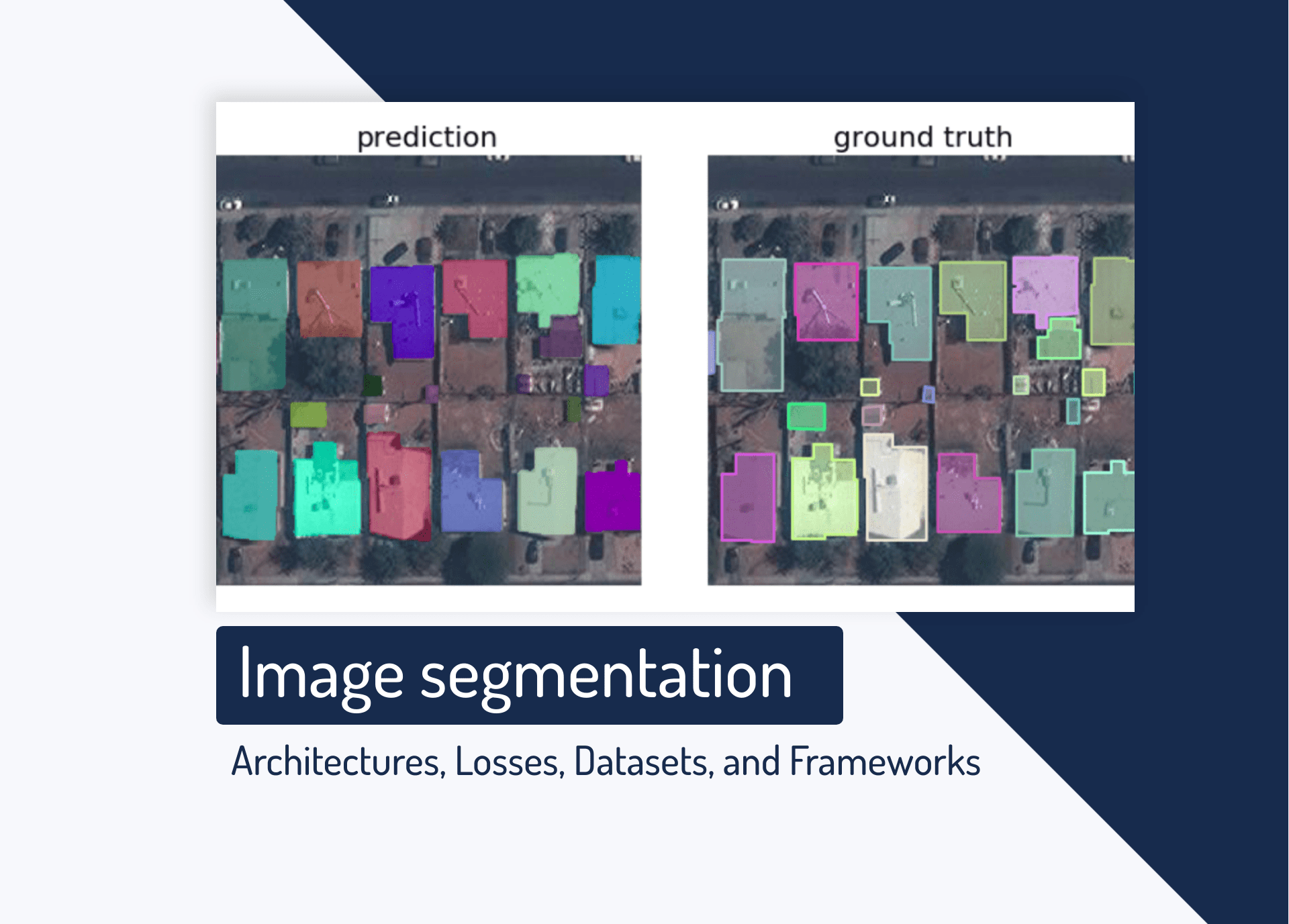In this piece, we’ll take a plunge into the world of image segmentation using deep learning. We’ll talk about:

• what image segmentation is

And the two main types of image segmentation

• Image segmentation architectures
• Loss functions used in image segmentation
• Frameworks that you can use for your image segmentation projects

## 1. What is Image Segmentation?

As the term suggests this is the process of dividing an image into multiple segments. In this process, every pixel in the image is associated with an object type. There are two major types of image segmentation — semantic segmentation and instance segmentation.

In semantic segmentation, all objects of the same type are marked using one class label while in instance segmentation similar objects get their own separate labels.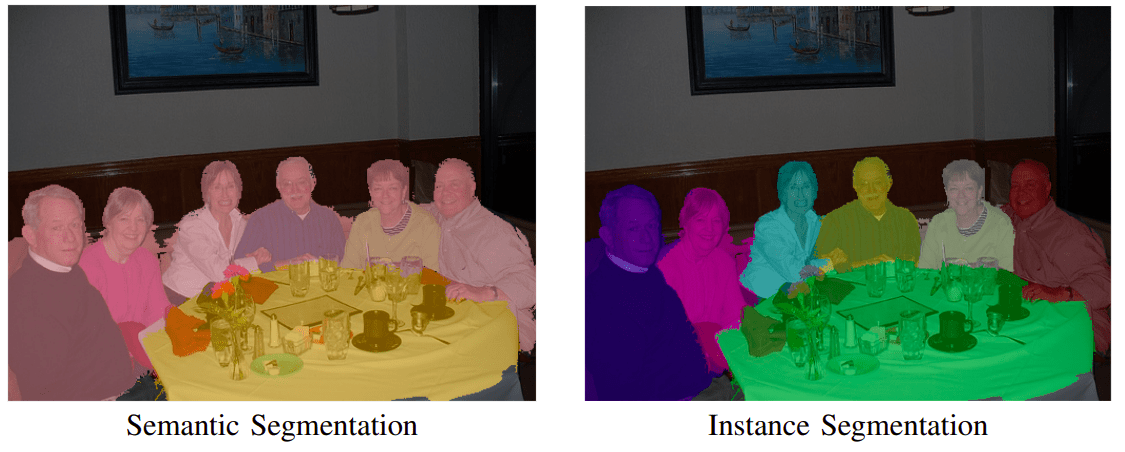Anurag Arnab, Shuai Zheng et. al 2018 “Conditional Random Fields Meet Deep Neural Networks for Semantic Segmentation” http://www.robots.ox.ac.uk/~tvg/publications/2017/CRFMeetCNN4SemanticSegmentation.pdf

## 2. Image Segmentation Architectures

The basic architecture in image segmentation consists of an encoder and a decoder.Vijay Badrinarayanan et. al 2017 “SegNet: A Deep Convolutional Encoder-Decoder Architecture for Image Segmentation” https://arxiv.org/abs/1511.00561

The encoder extracts features from the image through filters. The decoder is responsible for generating the final output which is usually a segmentation mask containing the outline of the object. Most of the architectures have this architecture or a variant of it.

### 2.1. U-Net

U-Net is a convolutional neural network originally developed for segmenting biomedical images. When visualized its architecture looks like the letter U and hence the name U-Net. Its architecture is made up of two parts, the left part — the contracting path and the right part — the expansive path. The purpose of the contracting path is to capture context while the role of the expansive path is to aid in precise localization.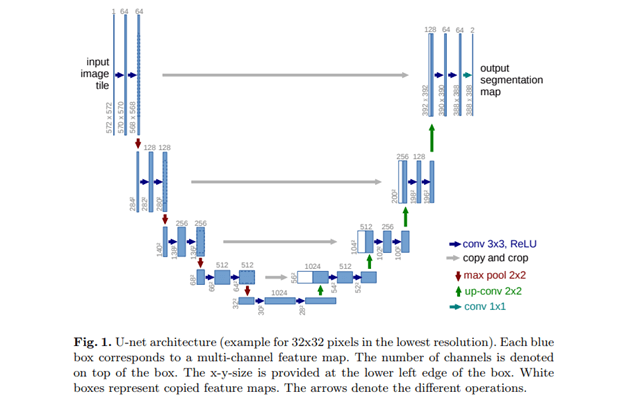Olaf Ronneberger et. al 2015 “U-net architecture image segmentation” https://arxiv.org/abs/1505.04597

U-Net is made up of an expansive path on the right and a contracting path on the left. The contracting path is made up of two three-by-three convolutions. The convolutions are followed by a rectified linear unit and a two-by-two max-pooling computation for downsampling.

U-Net’s full implementation can be found here.

https://lmb.informatik.uni-freiburg.de/people/ronneber/u-net/

### 2.2. FastFCN —Fast Fully-connected network

In this architecture, a Joint Pyramid Upsampling(JPU) module is used to replace dilated convolutions since they consume a lot of memory and time. It uses a fully-connected network at its core while applying JPU for upsampling. JPU upsamples the low-resolution feature maps to high-resolution feature maps.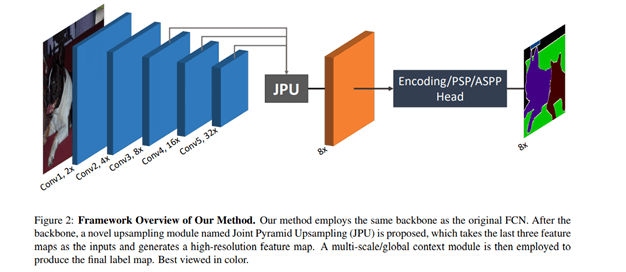Huikai Wu et.al 2019 “FastFCN: Rethinking Dilated Convolution in the Backbone for Semantic Segmentation” https://arxiv.org/abs/1903.11816

Github - FastFCN https://github.com/wuhuikai/FastFCN

### 2.3. Gated-SCNN

This architecture consists of a two-stream CNN architecture. In this model, a separate branch is used to process image shape information. The shape stream is used to process boundary information.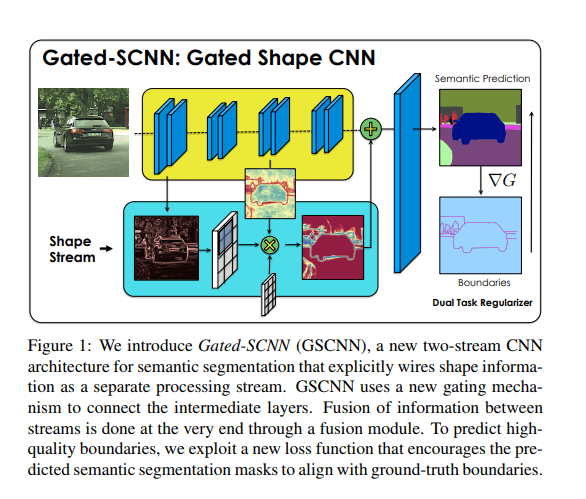Towaki Takikawa et. al 2019 “Gated-SCNN: Gated Shape CNNs for Semantic Segmentation” https://arxiv.org/abs/1907.05740

Github - GSCNN https://github.com/nv-tlabs/gscnn

### 2.4. DeepLab

In this architecture, convolutions with upsampled filters are used for tasks that involve dense prediction. Segmentation of objects at multiple scales is done via atrous spatial pyramid pooling. Finally, DCNNs are used to improve the localization of object boundaries. Atrous convolution is achieved by upsampling the filters through the insertion of zeros or sparse sampling of input feature maps.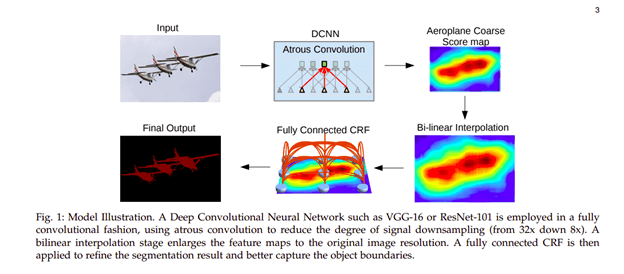Liang-Chieh Chen et. al 2016 “DeepLab: Semantic Image Segmentation with Deep Convolutional Nets, Atrous Convolution, and Fully Connected CRFs” https://arxiv.org/abs/1606.00915

Github - deeplabv3(PyTorch) https://github.com/fregu856/deeplabv3

Github - deeplab_v3(TensorFlow) https://github.com/sthalles/deeplab_v3

In this architecture, objects are classified and localized using a bounding box and semantic segmentation that classifies each pixel into a set of categories. Every region of interest gets a segmentation mask. A class label and a bounding box are produced as the final output. The architecture is an extension of the Faster R-CNN. The Faster R-CNN is made up of a deep convolutional network that proposes the regions and a detector that utilizes the regions.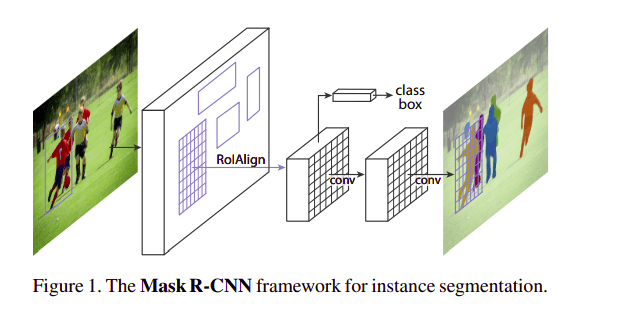Kaiming He et. al 2017 “Mask R-CNN” https://arxiv.org/abs/1703.06870

Here is an image of the result obtained on the COCO test set.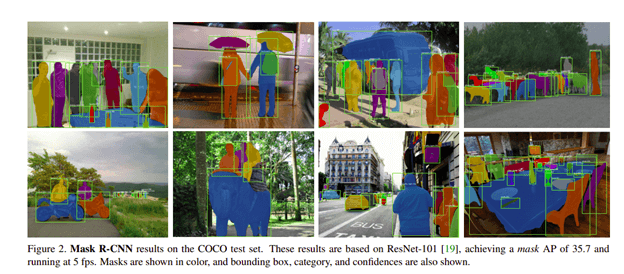## 3. Image Segmentation Loss functions

Semantic segmentation models usually use a simple cross-categorical entropy loss function during training. However, if you are interested in getting the granular information of an image, then you have to revert to slightly more advanced loss functions. ‘

### 3.1. Focal Loss

This loss is an improvement to the standard cross-entropy criterion. This is done by changing its shape such that the loss assigned to well-classified examples is down-weighted. Ultimately, this ensures that there is no class imbalance. In this loss function, the cross-entropy loss is scaled with the scaling factors decaying at zero as the confidence in the correct classes increases. The scaling factor automatically down weights the contribution of easy examples at training time and focuses on the hard ones.

$$FL(p_t) = - (1 - p_t)^{\gamma} log(p_t)$$

### 3.2. Dice Loss

This loss is obtained by calculating smooth dice coefficient function. This loss is the most commonly used loss is segmentation problems.

$$DSC = \frac{2|X \bigcap Y|}{|X| + |Y|}$$

### 3.3. Intersection over Union (IoU)-balanced Loss

The IoU-balanced classification loss aims at increasing the gradient of samples with high IoU and decreasing the gradient of samples with low IoU. In this way, the localization accuracy of machine learning models is increased.

$$IoU = \frac{TP}{(TP+FP+FN)}$$

### 3.4. Boundary Loss

One variant of the boundary loss is applied to tasks with highly unbalanced segmentations. This loss’s form is that of a distance metric on space contours and not regions. In this manner, it tackles the problem posed by regional losses for highly imbalanced segmentation tasks.

$$Dist(\partial G, \partial S) = \int _{\partial G } ||y \partial S(p) - p||^2 dp$$

### 3.5. Weighted cross-entropy

In one variant of cross-entropy, all positive examples are weighted by a certain coefficient. It is used in scenarios that involve class imbalance.

$$WCE(p, \hat{p}) = -(\beta p log(\hat{p}) + (1-p)log(1 - \hat{p}))$$

### 3.6. Lovász-Softmax loss

This loss performs direct optimization of the mean intersection-over-union loss in neural networks based on the convex Lovasz extension of sub-modular losses.

$$loss(f) = \frac{1}{|C|} \sum_{c \in C} \overline{\Delta J_c} (m (c))$$

Other losses worth mentioning are:

• TopK loss whose aim is to ensure that networks concentrate on hard samples during the training process.
• Distance penalized CE loss that directs the network to boundary regions that are hard to segment.
• Sensitivity-Specificity (SS) loss that computes the weighted sum of the mean squared difference of specificity and sensitivity.
• Hausdorff distance(HD) loss that estimated the Hausdorff distance from a convolutional neural network.

These are just a couple of loss functions used in image segmentation. To explore many more check out this repo.

Github - SegLoss https://github.com/JunMa11/SegLoss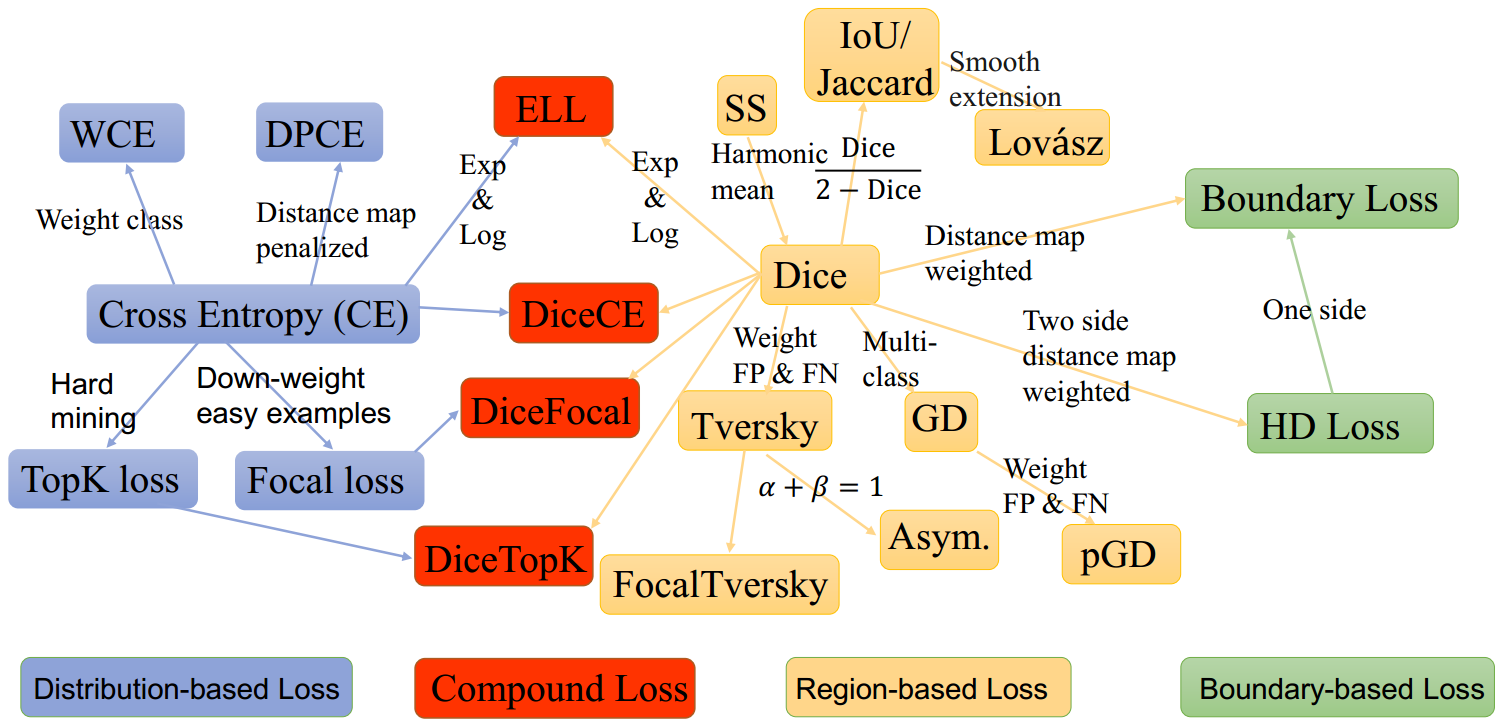## 4. Image Segmentation Datasets

If you are still here, chances are that you might be asking yourself where you can get some datasets to get started.

### 4.1. Common Objects in Context — Coco Dataset

COCO is a large-scale object detection, segmentation, and captioning dataset. The dataset contains 91 classes. It has 250,000 people with key points. Its download size is 37.57 GiB. It contains 80 object categories. It is available under the Apache 2.0 License and can be downloaded from here.

### 4.2. PASCAL Visual Object Classes (PASCAL VOC)

PASCAL has 9963 images with 20 different classes. The training/validation set is a 2GB tar file. The dataset can be downloaded from the official website.

### 4.3. The Cityscapes Dataset

This dataset contains images of city scenes. It can be used to evaluate the performance of vision algorithms in urban scenarios. The dataset can be downloaded from here.

### 4.4. The Cambridge-driving Labeled Video Database — CamVid

This is a motion-based segmentation and recognition dataset. It contains 32 semantic classes. This link contains further explanations and download links to the dataset.

## 5. Image Segmentation Frameworks

Now that you are armed with possible datasets, let’s mention a few tools/frameworks that you can use to get started.

• FastAI library— given an image this library is able to create a mask of the objects in the image.
• Sefexa Image Segmentation Tool— Sefexa is a free tool that can be used for Semi-automatic image segmentation, analysis of images, and creation of ground truth
• MultiPath — This a Torch implementation of the object detection network from “A MultiPath Network for Object Detection”.
• OpenCV — This is an open-source computer vision library with over 2500 optimized algorithms.
• MIScnn is a medical image segmentation open-source library. It allows setting up pipelines with state-of-the-art convolutional neural networks and deep learning models in a few lines of code.
• Fritz: Fritz offers several computer vision tools including image segmentation tools for mobile devices.

## 6. Final Thoughts

Hopefully, this article gave you some background into image segmentation and given you some tools and frameworks that you can use to get started.

We’ve covered:

• what image segmentation is,
• a couple of image segmentation architectures,
• some image segmentation losses,
• image segmentation tools and frameworks.